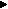## lørdag 22. september 2007

### TI-BASIC Programs: Binomial Distribution and Sum of Binomial Distributions

Note: This is NOT a programming tutorial, but rather a walk through intended to show you the development of a program. Therefore, I am not going to explain the code line for line but I will explain the purpose of the program and tell you shortly about how it works.

Even though I have written and tested this program on a TI-84 Plus calculator, it should work on any of Texas Instruments' graphing calculators as they all have the TI-BASIC language built into them.

If the only reason why you're reading this is because you need one of the programs, jump to the "Binomial Distribution" program code or jump to the "Sum of Binomial Distributions" program code. If you are here to read the whole thing, just keep on reading.

For more information about the TI-84 Plus calculator, download the TI-84 Plus Guide Books. In these guide books you will find more information about the different commands that I use in this program. For more on TI-BASIC, you should also check out the TI-BASIC Programming Wikibook, TI-Basic Developer, ticalc.org and the TI-83 Plus SDK documentation (works for TI-84 Plus as well).

Last month we worked with probability at school. Using our TI-84 Plus calculators and the formula for Binomial Distribution, we found the probability for r successes out of n trials to occur.

To find this you have to type the following on the calculator:

(n nCr r)*p^r*(1-p)^(n-r)

Where the letters which are underlined should be replaced by numbers.

This can be somewhat time consuming, so I decided to make a simple program (written in TI-BASIC) that would ask for n, r and p before it calculates the probability, thereby reducing the amount of keystrokes required to be performed by the user. Also, this program will give you the answer as a fraction (which often is an advantage when working with probability) where possible. Another advantage with my program is that it detects illegal values before the calculation has started. In other words, the purpose of this program is to save the user from having to perform unnecessarily time consuming operations. As you may understand, if the program was to ask for variables and then perform a calculation (which, depending on how fast the calculator is and how advanced the operation is, may take some time) only to break at the very end of an operation and give an error-message, it would in fact work against its purpose.

Program code: Binomial Distribution v1.0 aka. BINDISTR
`:Prompt N:Prompt R:While R>N:Disp "NO! R SHOULD BE":Disp "IN THE INTERVAL":Disp "WHERE R≤N":Prompt N:Prompt R:End:Prompt P:While not(0≤P and P≤1):Disp "NO! P SHOULD BE":Disp "IN THE INTERVAL":Disp "WHERE 0≤P≤1":Prompt P:End:ClrHome:Disp "THE ANSWER IS:":(N nCr R)*P^R*(1-P)^(N-R)Frac`

The next thing we learned about at school was finding the probability for r OR r+1 OR r+2 OR ... successes out of n trials to occur. In order to find this, you simply sum the all the probabilities for the different scenarios. This of course, is even more time consuming than the previous example, so I decided to modify the program so that it would ask for n, lowest r, highest r and p and then calculate the probability. I figured this would be a nice way to do that:

Program code: Sum of Binomial Distributions v1.0 aka. BINOMSUM
`:0→A:Prompt N:Disp "LOWEST R:":Prompt L:While L>N:Disp "NO! R SHOULD BE":Disp "IN THE INTERVAL":Disp "WHERE R≤N":Prompt N:Prompt L:End:Disp "HIGHEST R:":Prompt H:While H>N:Disp "NO! H SHOULD BE":Disp "IN THE INTERVAL":Disp "WHERE H≤N":Prompt H:End:While L>H:Disp "NO! H SHOULD BE":Disp "SO THAT L≤H":Prompt H:End:Prompt P:While not(0≤P and P≤1):Disp "NO! P SHOULD BE":Disp "IN THE INTERVAL":Disp "WHERE 0≤P≤1":Prompt P:End:While L≤H:((N nCr L)*P^L*(1-P)^(N-L)+A)→A):(L+1)→L:End:ClrHome:Disp "THE ANSWER IS:":AFrac`

The next day, my teacher told me that the calculator already has a method for summing a sequence of probabilities and the time taken by the calculator to calculate the probability using this algorithm proved to be faster than my method:

sum(seq(n nCr X*p^X*(1-p)^(n-X),X,l,h))

Where the letters which are underlined should be replaced by numbers.

However, once again this involves a lot of typing for the user which means unnecessary usage of time. Therefore I decided to implement the algorithm above in my program. I think that a change in the algorithm should be considered as a major change, therefore I change the version number to 2.0

Program code: Sum of Binomial Distributions v2.0 aka. BINOMSUM
`:Prompt N:Disp "LOWEST R:":Prompt L:While L>N:Disp "NO! R SHOULD BE":Disp "IN THE INTERVAL":Disp "WHERE R≤N":Prompt N:Prompt L:End:Disp "HIGHEST R:":Prompt H:While H>N:Disp "NO! H SHOULD BE":Disp "IN THE INTERVAL":Disp "WHERE H≤N":Prompt H:End:While L>H:Disp "NO! H SHOULD BE":Disp "SO THAT L≤H":Prompt H:End:Prompt P:While not(0≤P and P≤1):Disp "NO! P SHOULD BE":Disp "IN THE INTERVAL":Disp "WHERE 0≤P≤1":Prompt P:End:sum(seq((N nCr X)*P^X*(1-P)^(N-X),X,L,H))`

Is there anything you think was unclear, do you think something is wrong in these programs? In that case, write a comment and I'll see what I can do.